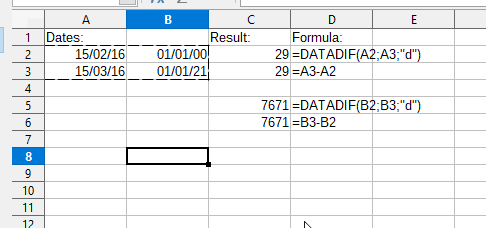Calc amount of days between dates [solved]

Hello. I have a simple problem but the answers which I have found, don’t work.
I have to calculate the number of days between two dates. Example:

A1=27-01-2010

A2=02-11-2011

C2=A2-A1 I can’t let it work.

A1 and A2 are correctly formatted as date DD-MM-YYYY

What just worked for me was to format the cells before entering the dates. When I typed in the dates first, and then applied the formats over top of them, I got #VALUE! from the formula. Format then data entry gave me 644.

Thank you, it seems to work!
Another detail is that the destination cell, where the amount of days is calculated (C2 here), has to be formatted as number and not as date.

It is best to use the DATEDIF function

DATEDIF() is unnecessary.

#VALUE! happens if one of the operands is not a numeric date but text content.

DATEDIF() gives me Err:511 for the same dates that subtraction works for.

In Portuguese DATADIF, in English DATEDIF“d” = Number of whole days between Start date and End date.

“m” = Number of whole months between Start date and End date.

“y” = Number of whole years between Start date and End date.

“ym” = Number of whole months when subtracting years from the difference of Start date and End date.

“md” = Number of whole days when subtracting years and months from the difference of Start date and End date.

“yd” = Number of whole days when subtracting years from the difference of Start date and End date.

I must be doing something else wrong, then, because I still get errors. Thanks for the interval information; I just found it in Help, too.

Actually, Help examples only show DATEDIF() used in formulas with the dates also in the formulas, not in other cells. I wonder if that’s the issue. Can you only have DATEDIF("2020-03-01";"2020-02-01";"d") and not DATEDIF(A2;A1;"d") where A2 and A1 contain dates?

Maybe someone would kindly add a “datedif” tag to this thread?@Inclement, example…

datedif.ods

Oh ack. I had the end date cell reference first like a dummy.
Thank you, @schiavinatto.

By the way, what I said up above is wrong, yes you can use cell references. It’s too late to edit that out now.

How to get number of working days only? between two dates. For instance, I would like to exclude Saturdays and Sundays as holidays.